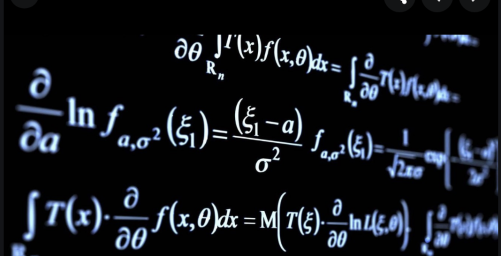Course

# Basic Maths for Data Science

Updated Mar 04, 2021

This is the crash course on #mathematics​. You will learn the very basic which will cover the fundamental foundation of mathematics. In this comprehensive tutorial you will learn about the following topic:

Consumer math - Percents

Consumer math - Interest

Consumer math - Mortgages

Consumer math - Inflation

Probability and statistics - Introduction to probability

Probability and statistics - Dependent and independent probabilities

Probability and statistics - Conditional probabilities

Probability and statistics - Expected values

Probability and statistics - introduction to statistics

Probability and statistics - Normal curves

Probability and statistics - Fraud detection

;